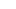# Top Strategies for Enhancing Data Frames: A Guide to Adding Empty Columns in R

Data frames are one of the most powerful and versatile data structures in R, making it easy to store, manipulate, and analyze structured data. They are similar to tables in a relational database, with columns representing variables and rows representing observations.

## The Importance of Empty Columns

Sometimes, while working with data frames, you may need to add an empty column for a variety of reasons. For instance, you may want to create a placeholder for derived or aggregated data or to store intermediate results during data processing. In this article, we will explore how to add empty column to dataframe in R, making your life easier when it comes to data manipulation.

## Methods to Add an Empty Column to a Data Frame in R

We will cover three different methods that can be used to add an empty column to a data frame in R:

### Method 1: The \$ Operator

The \$ operator is the simplest way to add an empty column to a data frame in R. Here’s a step-by-step guide:

2. Use the \$ operator followed by the name of the new column.
3. Assign a vector of NA values with the same length as the number of rows in the data frame.

Example:

data_frame\$new_column <- rep(NA, nrow(data_frame))

### Method 2: The cbind() Function

The cbind() function can also be used to add an empty column to a data frame. Here’s a step-by-step guide:

2. Use the cbind() function to combine the data frame with a new column.
3. Assign a vector of NA values with the same length as the number of rows in the data frame.

Example:

data_frame <- cbind(data_frame, new_column = rep(NA, nrow(data_frame)))

### Method 3: The dplyr Package

The dplyr package is a popular package for data manipulation in R, and it provides a function called mutate() that can be used to add an empty column. Here’s a step-by-step guide:

1. Install and load the dplyr package.
3. Use the mutate() function followed by the name of the new column.
4. Assign a vector of `NA values with the same length as the number of rows in the data frame.

Example:

library(dplyr)

data_frame <- data_frame %>%

mutate(new_column = rep(NA, nrow(data_frame)))

## Comparing Methods: Pros and Cons

Each of the methods discussed above has its own advantages and disadvantages. Let’s compare them:

### The \$ Operator

Pros:

• Simple and straightforward.
• Does not require additional packages.

Cons:

• Limited flexibility, as it can only add a single column at a time.

### The cbind() Function

Pros:

• Can add multiple columns at once.
• No need for additional packages.

Cons:

• Can be less efficient for large data frames, as it creates a new data frame during the process.

Pros: